# Doc: Continuity of a Function JEE Notes | EduRev

## JEE : Doc: Continuity of a Function JEE Notes | EduRev

The document Doc: Continuity of a Function JEE Notes | EduRev is a part of the JEE Course Mathematics (Maths) Class 12.
All you need of JEE at this link: JEE

A. Definition of Continuity

Continuity at a Point: A function f is continuous at c if the following three conditions are met.
(i) f(x) is defined.
(ii)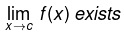(iii)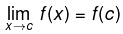In other words function f(x) is said to be continuous at x = c , if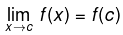Symbolically f is continuous at  x = c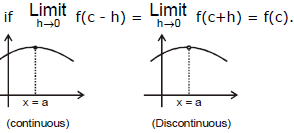One-sided Continuity:

• A function f defined in some neighbourhood of a point c for c ⇒ c is said to be continuous at c from the left if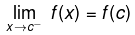• A function f defined in some neigbourhood of a point c for x ³ c is said to be continuous at c from the right if• One-sided continuity is a collective term for functions continuous from the left or from the right.
• If the function f is continuous at c, then it is continuous at c from the left and from the right . Conversely, if the function f is continuous at c from the left and from the right, then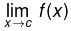exists &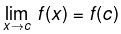• The last equality means that f is continuous at c.
• If one of the one-sided limits does not exist, thendoes not exist either. In this case, the point c is a discontinuity in the function, since the continuity condition is not met.

Continuity In An Interval
(a) A function f is said to be continuous in an open interval (a , b) if f is continuous at each & every point ∈(a, b).
(b) A function f is said to be continuous in a closed interval [a,b] if:

• f is continuous in the open interval (a , b) &
• f is right continuous at `a' i.e.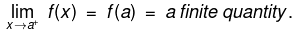• f is left continuous at `b' i.e.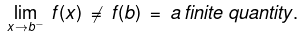A function f can be discontinuous due to any of the following three reasons :

•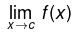does not exist i.e.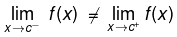• f(x) is not defined at x= c
•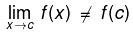• Geometrically, the graph of the function will exhibit a break at x= c.

Example 1. Test the following functions for continuity
(a) 2x5 - 8x2 + 11 / x4 + 4x3 + 8x2 + 8x +4
(b) f(x) = 3sin3x + cos2x + 1 / 4cos x - 2
Solution
(a) A function representing a ratio of two continuous functions will be (polynomials in this case) discontinuous only at points for which the denominator zero. But in this case (x4 + 4x3 + 8x2 + 8x + 4) = (x2 + 2x + 2)2 = [(x + 1)2 + 1]2 > 0 (always greater than zero)
Hence f(x) is continuous throughout the entire real line.
(b) The function f(x) suffers discontinuities only at points for which the denominator is equal to zero i.e. 4 cos x - 2 = 0 or cos x = 1/2 ⇒ x = xn = ± π/3 + 2nπ(n=0, ±1, ±2...) Thus the function f(x) is continuous everywhere, except at the point xn.

Example 2.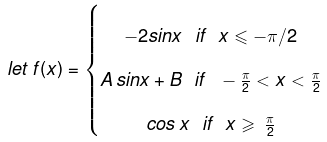Find A and B so as to make the function continuous.
SolutionAt x =  - π/2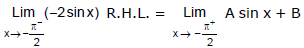- π/2 - h
where h→ 0
Replace x by - π/2+h
where h → 0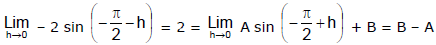So B - A = 2 ...(i)
At x = π/2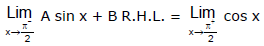Replace x by π/2 - h
Replace x by π/2+h
where h→ 0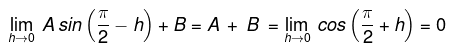So    A+B = 0      ...(ii)
Solving (i) & (ii),    B= 1, A = -1

Example 3. Test the continuity of f(x) at x = 0 if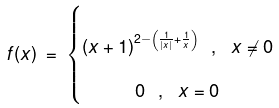SolutionFor x < 0,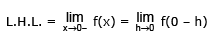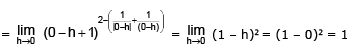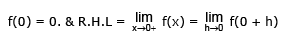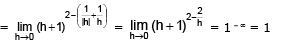L.H.L. = R.H.L. ≠ f(0) Hence f(x) is discontinuous at x = 0.

Example 4. If f(x) be continuous function for all real values of x and satisfies;
x2 + {f(x) – 2} x + 2√3 – 3 – √3 . f(x) = 0, for  x ∈ R. Then find the value of f(√3 ).
Solution. As f(x) is continuous for all x ∈ R.
Thus,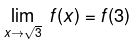where
f(x) = x2 - 2x + 2√3 - 3 / √3 - x, x ≠ √3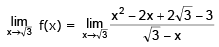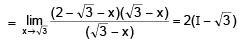f(√3) = 2(1-√3).

Example 5.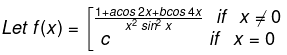If f (x) is continuous at x = 0, then find the value  of (b+c)3-3a.
Solution.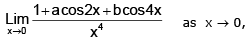N→ 1 + a + b D→ 0
for existence of limit a + b + 1 = 0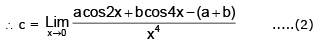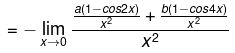limit of Nr ⇒ 2a+8b = 0 ⇒ a = -4b
hence
-4b+b = -1
⇒ b = 1/3 and a = -4/3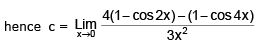= 8 sin2x - 2 sin22x / 3x4 = 8 sin2x - 8sin2xcos2x / 3x4

= 8 / 3 . sin2x / x2 . sin2x / x2 = 8 / 3

⇒ eA = 1 / 2 (e2x A / x + B / x) ⇒ x . eA = 1 / 2 (e2x . A + B)

Example 6.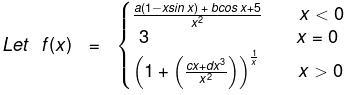If f is continuous at x = 0, then find the values of a, b, c & d.
Solution.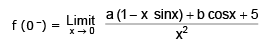,
for existence of limit  a + b + 5 = 0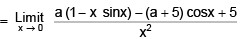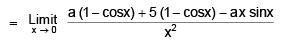= a / 2 + 5 / 2 - a = 3
⇒ a = - 1 ⇒ b = - 4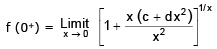for existence of limit  c = 0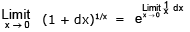= ed = 3 ⇒ d = ln 3

Example 7. Let f(x) = x3 = 3x2 + 6 ∀ x ∈ R and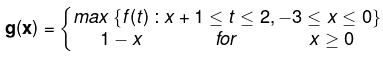Test continuity of g (x) for  x ∈ [-3, 1].
Solution. Since f(x) = x3 - 3x2 + 6  ⇒ f'(x) = 3x2 - 6x = 3x (x - 2) for maxima and minima f'(x) = 0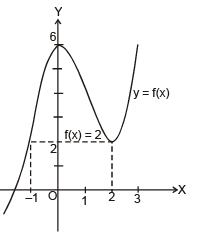x = 0, 2
f"(x) = 6x – 6
f" (0) = –6 < 0 (local maxima at x = 0)
f" (2) = 6 > 0 (local minima at x = 2)
x3 – 3x2 + 6 = 0 has maximum 2 positive and 1 negative real roots. f(0) = 6.
Now graph of f(x) is :
Clearly f(x) is increasing in (– ∝, 0) U (2, ∝) and decreasing in (0, 2)
⇒ x + 2 < 0 ⇒ x < – 2 ⇒ –3 ≤ x < – 2
⇒ –2 ≤ x + 1 < –1 and –1 ≤ x + 2 < 0
in both cases f(x) increases (maximum) of g(x) = f(x + 2)
g(x) = f(x + 2); –3 ≤ x < – 2 ...(1)
and if x + 1 < 0 and 0 ≤ x + 2 < 2
– 2 ≤ x < –1 then g(x) = f(0)
Now for x + 1 ≥ 0 and x + 2 < 2 ⇒ –1 ≤ x < 0, g(x) = f(x + 1)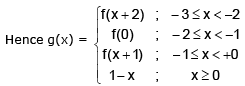Hence g(x) is continuous in the interval [–3, 1].

Example 8. Given the function,
f(x) = x [ 1 / x(1 + x) + 1 / (1 + x)(1 + 2x) + 1 / (1 + 2x)(1 + 3x) + ....upto ∞

Find f (0) if f (x) is continuous at x = 0.
Solution.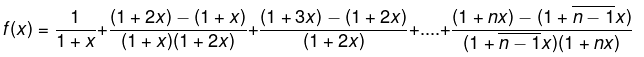f(x) = 2 / 1 + x - 1 / 1 + nx upto n terms when x ≠0.
Hence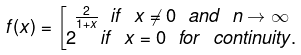Example 9.  Let f: R →R be a function which satisfies f(x+y3) = f(x) + (f(y))3 ∀ x, y ∈ R. If f is continuous at x = 0, prove that f is continuous every where.
Solution.
To prove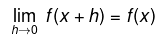Put x = y = 0 in the given relation f(0) = f(0) + (f(0))3 ⇒ f(0) = 0
Since f is continuous at x = 0
To prove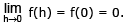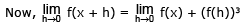= f(x) + 0 = f(x).

Hence f is continuous for all x ∈ R.

Offer running on EduRev: Apply code STAYHOME200 to get INR 200 off on our premium plan EduRev Infinity!

## Mathematics (Maths) Class 12

209 videos|222 docs|124 tests

,

,

,

,

,

,

,

,

,

,

,

,

,

,

,

,

,

,

,

,

,

;